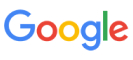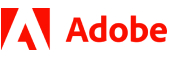New update is available. Click here to update.
Topics

# Intersection Of Two Sorted Arrays

Easy0/40
Average time to solve is 10m+15 more companies

## Problem statement

You are given two arrays 'A' and 'B' of size 'N' and 'M' respectively. Both these arrays are sorted in non-decreasing order. You have to find the intersection of these two arrays.

Intersection of two arrays is an array that consists of all the common elements occurring in both arrays.

Note :
``````1. The length of each array is greater than zero.
2. Both the arrays are sorted in non-decreasing order.
3. The output should be in the order of elements that occur in the original arrays.
4. If there is no intersection present then return an empty array.
``````
Detailed explanation ( Input/output format, Notes, Images )
Constraints :
``````1 <= T <= 100
1 <= N, M <= 10^4
0 <= A[i] <= 10^5
0 <= B[i] <= 10^5

Time Limit: 1 sec
``````
Sample Input 1 :
``````2
6 4
1 2 2 2 3 4
2 2 3 3
3 2
1 2 3
3 4
``````
Sample Output 1 :
``````2 2 3
3
``````
Explanation for Sample Input 1 :
``````For the first test case, the common elements are 2 2 3 in both the arrays, so we print it.

For the second test case, only 3 is common so we print 3.
``````
Sample Input 2 :
``````2
3 3
1 4 5
3 4 5
1 1
3
6
``````
Sample Output 2 :
``````4 5
-1
``````Console